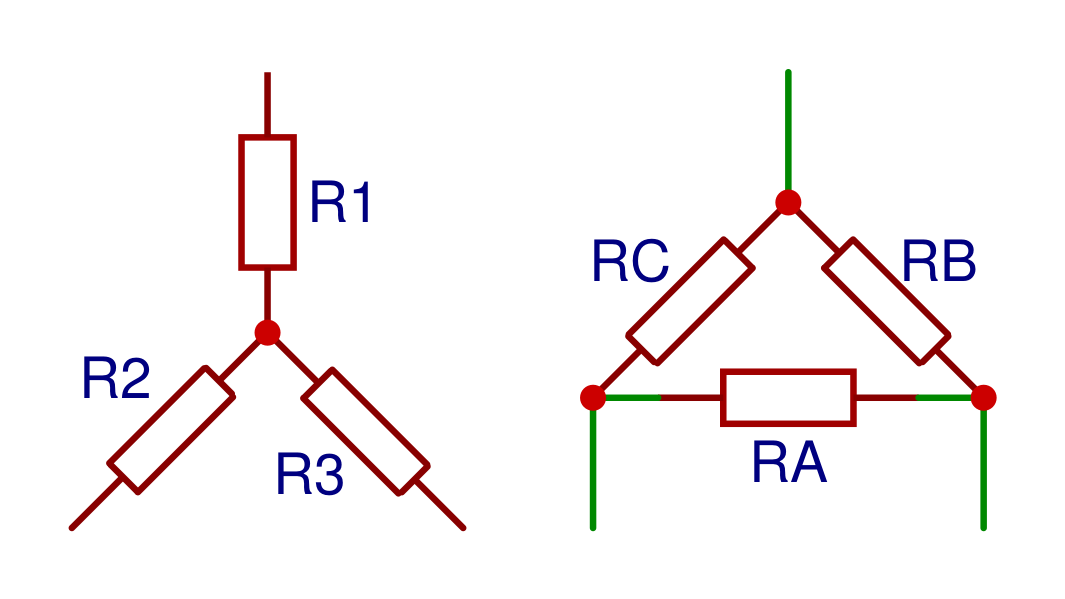# star delta transformation

The Y-Δ (wye-delta) and Δ-Y (delta-wye) transformations are used to calculate the resistances in the corresponding Δ or Y circuit. These circuits consist of three resistors connected as in the image below.

### Formulas

#### Star to delta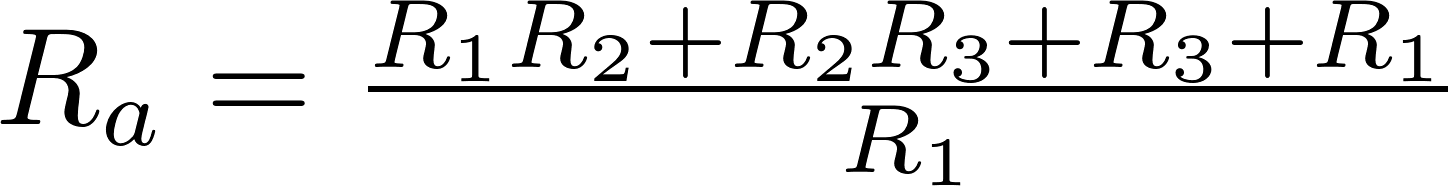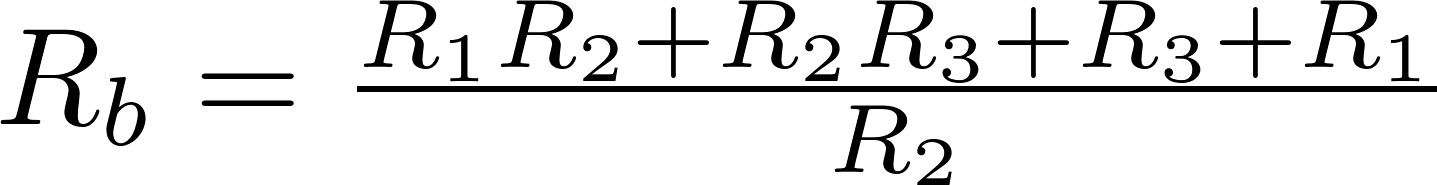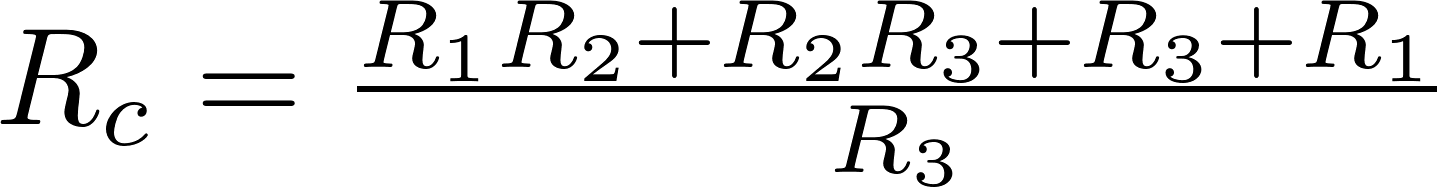#### Delta to star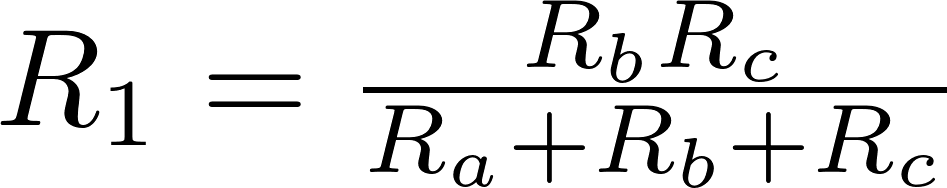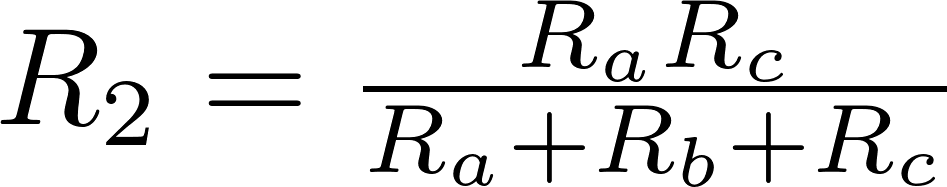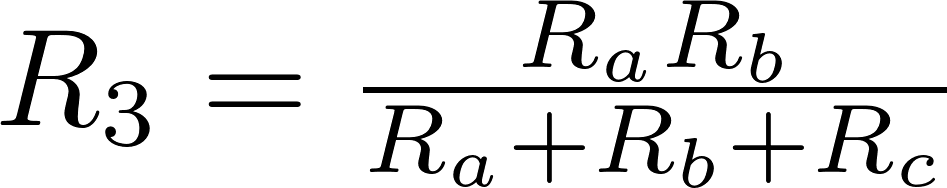R is the symbol for resistance and is measured in ohm (Ω).